# C语言结构体数组的直接赋值及数组的长度计算

16 篇文章 1 订阅

typedef struct Pi {
int x;
int y;
} Pc;

int main() {
int i;
Pc pc;
pc.x = 1;
pc.y = 2;
pc.x = 3;
pc.y = 4;
for (i = 0; i < 2; i++) {
printf("x=%d  y=%d\n", pc[i].x, pc[i].y);
}
return 0;
}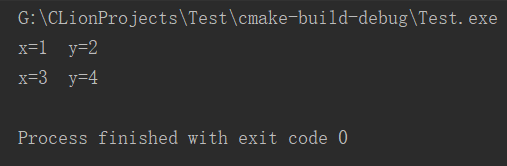typedef struct Pi {
int x;
int y;
} Pc;

int main() {

Pc pc = {
.x = 3,
.y = 4,
.x = 5,
.y = 6
};
for (int i = 0; i < 2; i++) {
printf("x=%d  y=%d\n", pc[i].x, pc[i].y);
}
return 0;
}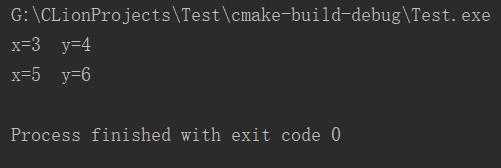#include <stdio.h>

//自定义一个字符串的结构体，包含字符串和字符串长度两个变量
typedef struct Str {
char ch;
int length;//char数组(字符串)的长度
} myStr;

//刚开始声明变量时每个变量的字符串长度length都为0
//这里以长度为10的数组为例，若数组长度是1000，一个一个赋值就累死了
//对第0个到第9个结构体数组的长度同时赋值为0
myStr myStr1 = {
[0 ... 9] = {
.length = 0,
}
};

int main() {
int i;
for (i = 0; i < 10; i++) {
printf("%d\n", myStr1[i].length);
}
return 0;
}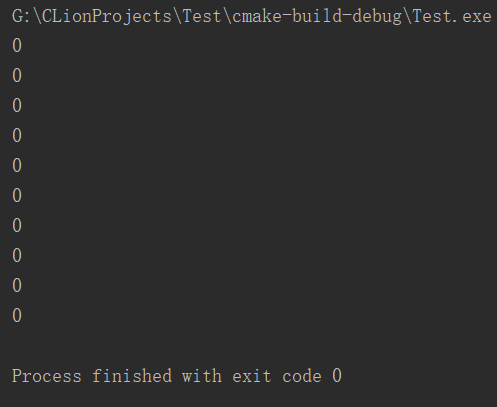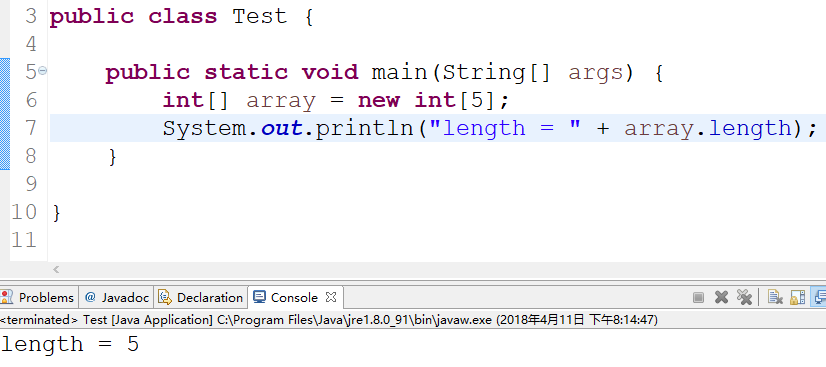int main() {
int  a[]={1,2,3,4,5,6,7,8};
//int类型数组a的总空间除以int类型的变量所占的空间就是数组的元素个数(长度)
int len=sizeof(a)/sizeof(int);
printf("length = %d\n",len);
return 0;
}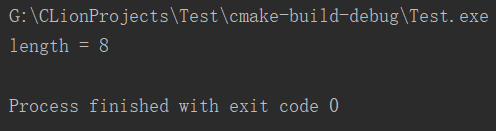typedef struct Pi {
int x;
int y;
} Pc;

int main() {
Pc pc = {
.x = 3,
.y = 4,
.x = 5,
.y = 6
};

//sizeof(pc)是pc所包含的所有属性所占的空间
printf("Total = %d\n", sizeof(pc));

/*
* 2 * sizeof(int)是一个pc结构体类型的变量所占的空间，
* 因为pc的结构体中包含两个int类型的属性
*/
//总的空间除以每个结构体所占的空间，也就是数组的长度
int len = sizeof(pc) / (2 * sizeof(int));
printf("length = %d\n", len);

//输出
for (int i = 0; i < len; ++i) {
printf("x=%d  y=%d\n", pc[i].x, pc[i].y);
}
}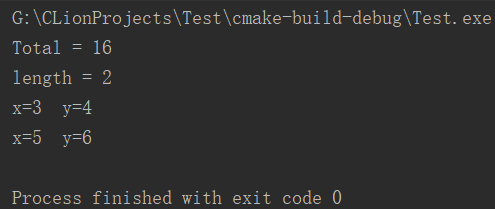int main() {
int array[] = {1, 2, 3, 4, 5, 6, 7, 8};
/*
* 数组长度：
* 数组占内存总空间，除以单个元素占内存空间大小
*/
int length = sizeof(array) / sizeof(array);
printf("length = %d", length);
}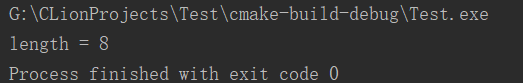int getLength(int array[]){
//这样求长度是错误的，得到的length的结果永远是1
int length = sizeof(array) / sizeof(array);
return length;
}

int main() {
int array[] = {1, 2, 3, 4, 5, 6, 7, 8};
int length = getLength(array);
printf("length = %d", length);
}

ps：因为在getLength()函数中,array是函数参数，到了该函数中，array只是一个指针(地址，系统在该函数运行时，是不知道array所表示的地址有多大的数据存储空间，这里只是告诉该函数一个数据存储空间首地址)，所以，sizoef(array)的结果是指针变量array占内存的大小，一般是4个字节。array是int类型，sizeof(array)也是4个字节，所以，结果永远是1。因此，获得数组长度，只能在数组定义所在的代码区中，采用上述简便方法，才可以达到效果。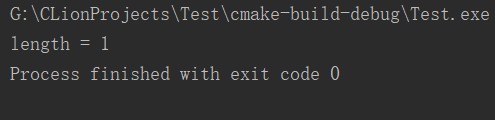08-28
02-0113706-044万+
11-29162
01-037万+
05-21970
10-12505
03-126113
08-03108
05-0416万+
10-29974
06-195188
04-164万+
07-052656
07-314878点击重新获取扫码支付余额充值